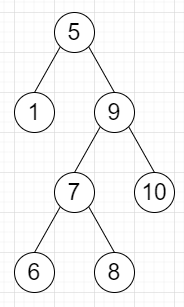# Program to check whether one value is present in BST or not in Python

Suppose we have a binary search tree and another input called val, we have to check whether val is present in the tree or not.

So, if the input is likeval = 7, then the output will be True, as 7 is present in the tree.

To solve this, we will follow these steps−

• Define a function solve() . This will take root, val

• if root is null, then

• return False

• if data of root is same as val, then

• return True

• if data of root < val, then

• return solve(left of root, val)

• return solve(right of root, val)

Let us see the following implementation to get better understanding−

Live Demo

## Example

class TreeNode:
def __init__(self, data, left = None, right = None):
self.data = data
self.left = left
self.right = right
class Solution:
def solve(self, root, val):
if not root:
return False
if root.data == val:
return True
if root.data > val:
return self.solve(root.left, val)
return self.solve(root.right, val)
ob = Solution()
root = TreeNode(5)
root.left = TreeNode(1)
root.right = TreeNode(9) root.right.left = TreeNode(7) root.right.right = TreeNode(10) root.right.left.left = TreeNode(6) root.right.left.right = TreeNode(8) print(ob.solve(root, 7))

## Input

root = TreeNode(5)
root.left = TreeNode(1)
root.right = TreeNode(9)
root.right.left = TreeNode(7)
root.right.right = TreeNode(10)
root.right.left.left = TreeNode(6)
root.right.left.right = TreeNode(8)
7

## Output

True